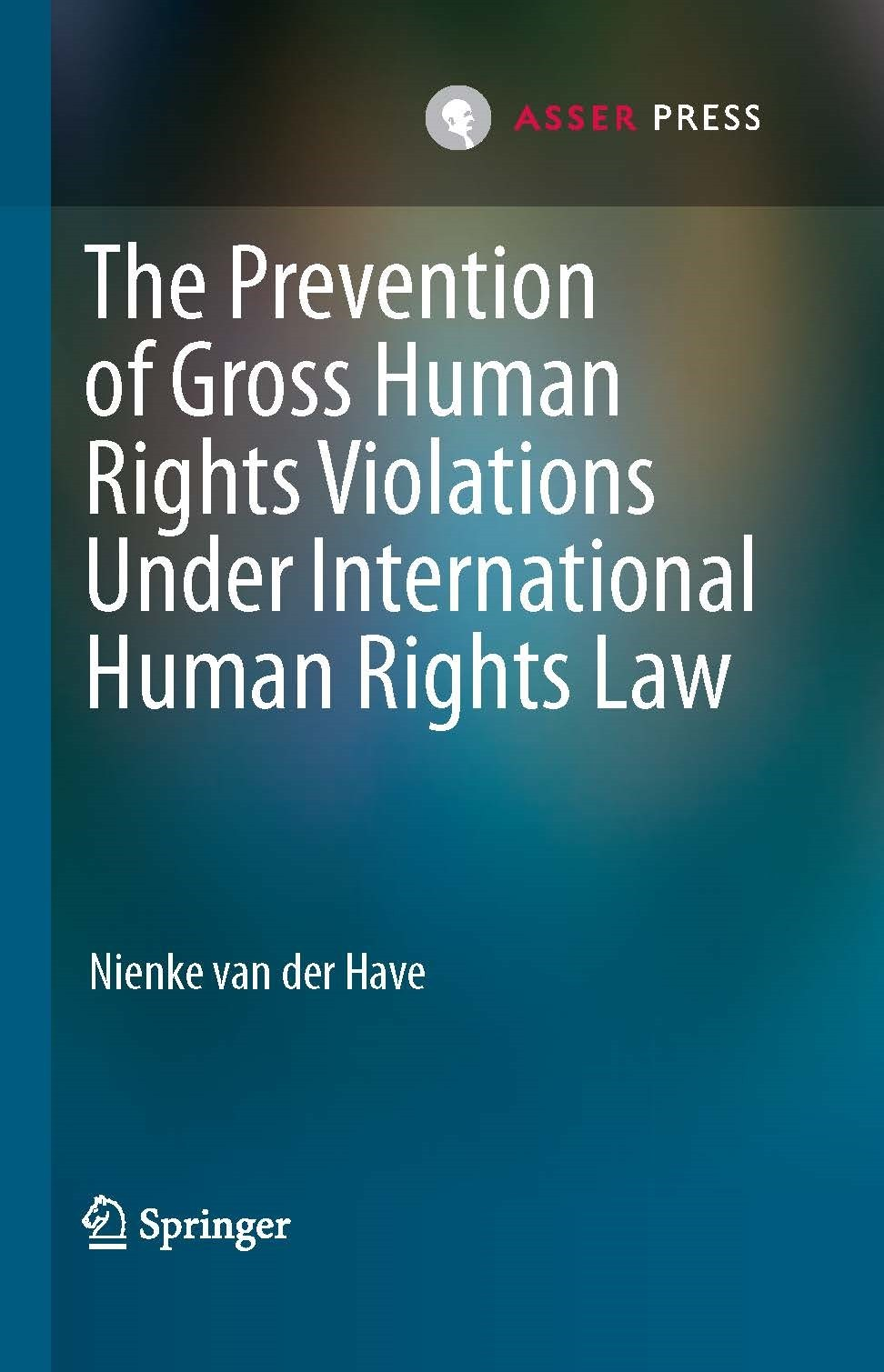Like   Tweet   in

# Asser Today January 2018

 table div table+table+table div table{width:100%;padding:0}table div table+table+table div table img{width:96.23%;padding:0;float:none}table div table+table+table div table td{width:100%;padding:0 1.88% 18px}/* styles */# Concluding Asser - Verfassungsblog Symposium: Final Thoughts on Mnemonic Constitutionalism

 /* styles */

## Selected Publications

▪ Berenice Boutin, Attribution of Conduct in International Military Operations: A Causal Analysis of Effective Control, 18(2) Melbourne Journal of International Law (2018), pp 154–179.
 ▪ Berenice Boutin, Attribution of Conduct in International Military Operations: A Causal Analysis of Effective Control, 18(2) Melbourne Journal of International Law (2018), pp 154–179.

▪ Christophe Paulussen and Kate Pitcher, Prosecuting (Potential) Foreign Fighters: Legislative and Practical Challenges, ICCT Research Paper.
 ▪ Christophe Paulussen and Kate Pitcher, Prosecuting (Potential) Foreign Fighters: Legislative and Practical Challenges, ICCT Research Paper.

 table div table+table+table+table+table+table+table+table+table+table+table+table div table{width:100%;padding:0}table div table+table+table+table+table+table+table+table+table+table+table+table div table img{width:96.23%;padding:0;float:none}table div table+table+table+table+table+table+table+table+table+table+table+table div table td{width:100%;padding:0 1.88% 18px}/* styles */# Education and Events

The Asser Institute has extensive experience in delivering postgraduate training programmes and in knowledge dissemination in the field of international and European law.

## Upcoming

 table div table+table+table+table+table+table+table+table+table+table+table+table+table+table div table{width:100%;padding:0}table div table+table+table+table+table+table+table+table+table+table+table+table+table+table div table img{width:96.23%;padding:0;float:none}table div table+table+table+table+table+table+table+table+table+table+table+table+table+table div table td{width:100%;padding:0 1.88% 18px}/* styles */table div table+table+table+table+table+table+table+table+table+table+table+table+table+table+table div table,table.module-14{width:43.77%;float:right;padding:0}table div table+table+table+table+table+table+table+table+table+table+table+table+table+table+table div table a{border:0 none;text-decoration:none}table div table+table+table+table+table+table+table+table+table+table+table+table+table+table+table div table img{width:100%!important;border:0 none;text-decoration:none}table div table+table+table+table+table+table+table+table+table+table+table+table+table+table+table div table td{width:100%;padding:0 0 20px 20px}/* styles */ 7 February Launch of the book ‘Legal Responses to Transnational and International Crimes. Towards an Integrative Approach’, followed by a Panel Discussion This book, co-edited by Prof. Harmen van der Wilt (University of Amsterdam) and Dr. Christophe Paulussen (Senior Researcher at the T.M.C. Asser Instituut), critically reflects on the connection between the ‘core’ crimes of the ICC, such as war crimes, and several newly emerging transnational crimes. During the launch, which is co-organised by the T.M.C. Asser Instituut, the Cassese Initiative and the IHCL Platform, both a more general presentation and a specific case study, on piracy, will be provided. The launch will start with a key-note speech by the President of Eurojust. More information and registration
 table div table+table+table+table+table+table+table+table+table+table+table+table+table+table+table+table div table,table.module-15{width:58.68%;float:left;padding:0}table div table+table+table+table+table+table+table+table+table+table+table+table+table+table+table+table div table a{border:0 none;text-decoration:none}table div table+table+table+table+table+table+table+table+table+table+table+table+table+table+table+table div table img{width:100%!important;border:0 none;text-decoration:none}table div table+table+table+table+table+table+table+table+table+table+table+table+table+table+table+table div table td{width:100%;padding:0 20px 20px 0}/* styles */ 14 February EU-Russia Relations in an Era of Sanctions This first CLEER lecture will be delivered by Prof. Peter van Elsuwege (University of Gent). It will take stock of the current relationship between the EU and Russia. Specific attention will be devoted to the legal and political implications of the sanctions regime. In addition, the future of EU-Russia relations will be discussed in light of the EU’s Global Strategy and pending challenges such as the evolution of the Eurasian Economic Union and the EU’s enlargement process towards the Western Balkans. More information and registration
 table div table+table+table+table+table+table+table+table+table+table+table+table+table+table+table+table+table div table,table.module-16{width:57.55%;float:right;padding:0}table div table+table+table+table+table+table+table+table+table+table+table+table+table+table+table+table+table div table a{border:0 none;text-decoration:none}table div table+table+table+table+table+table+table+table+table+table+table+table+table+table+table+table+table div table img{width:100%!important;border:0 none;text-decoration:none}table div table+table+table+table+table+table+table+table+table+table+table+table+table+table+table+table+table div table td{width:100%;padding:0 0 20px 20px}/* styles */ 20 February The International Court of Justice & Nuclear Weapons In October 2016, the ICJ delivered judgments in the case of “Obligations concerning Negotiations relating to Cessation of the Nuclear Arms Race and to Nuclear Disarmament” - Marshall Islands v. the United Kingdom, India and Pakistan, the three states that remained out of the nine states, against which the Marshall Islands had filed Applications. The Marshall Islands contended that the nuclear powers did not fulfill their obligations under Article VI of the Non-Proliferation Treaty ‘to pursue negotiations in good faith on effective measures relating to […] nuclear disarmament’. The very notion of this provision figured prominently in the ICJ’s 1996 Advisory Opinion on the “Legality of the Threat or Use of Nuclear Weapons” at the request of the General Assembly of the United Nations. The event will deal with the history of the Case and the Advisory Opinion (AO), as well as the relevant issues of international law and arms control law. More information and registration

# Highlight

 table div table+table+table+table+table+table+table+table+table+table+table+table+table+table+table+table+table+table+table div table{width:100%;padding:0}table div table+table+table+table+table+table+table+table+table+table+table+table+table+table+table+table+table+table+table div table img{width:96.23%;padding:0;float:none}table div table+table+table+table+table+table+table+table+table+table+table+table+table+table+table+table+table+table+table div table td{width:100%;padding:0 1.88% 18px}/* styles */## International and Transnational Criminal Law Training Course Marks New Asser - Cassese Initiative Cooperation

From 5 to 9 February 2018, the Asser Institute and the Antonio Cassese Initiative will host a training course on international and transnational criminal law (ICL and TCL) for judges and prosecutors from francophone African countries, including Mali, Cote d’Ivoire, Congo, Burkina Faso, Niger and the Democratic Republic of the Congo.

This training course marks the beginning of increased cooperation between the Asser Institute and the Antonio Cassese Initiative, which aims at setting up a permanent training facility on ICL and TCL for magistrates coming from countries facing challenges in the administration of justice. This permanent facility seeks to become the go-to venue for prosecutorial and judicial training courses regarding international and transnational crimes, as these concepts are increasingly intertwined.

 table div table+table+table+table+table+table+table+table+table+table+table+table+table+table+table+table+table+table+table+table+table div table{width:100%;padding:0}table div table+table+table+table+table+table+table+table+table+table+table+table+table+table+table+table+table+table+table+table+table div table img{width:96.23%;padding:0;float:none}table div table+table+table+table+table+table+table+table+table+table+table+table+table+table+table+table+table+table+table+table+table div table td{width:100%;padding:0 1.88% 18px}/* styles */table.module-21{width:43.21%;padding:0}table div table+table+table+table+table+table+table+table+table+table+table+table+table+table+table+table+table+table+table+table+table+table div table{width:43.21%;float:none;margin-left:auto;margin-right:auto;padding:0}table div table+table+table+table+table+table+table+table+table+table+table+table+table+table+table+table+table+table+table+table+table+table div table a{border:0 none;text-decoration:none}table div table+table+table+table+table+table+table+table+table+table+table+table+table+table+table+table+table+table+table+table+table+table div table img{width:100%!important;border:0 none;text-decoration:none}table div table+table+table+table+table+table+table+table+table+table+table+table+table+table+table+table+table+table+table+table+table+table div table td{width:100%;padding:0}/* styles */
 table div table+table+table+table+table+table+table+table+table+table+table+table+table+table+table+table+table+table+table+table+table+table+table div table{width:100%;padding:0}table div table+table+table+table+table+table+table+table+table+table+table+table+table+table+table+table+table+table+table+table+table+table+table div table img{width:96.23%;padding:0;float:none}table div table+table+table+table+table+table+table+table+table+table+table+table+table+table+table+table+table+table+table+table+table+table+table div table td{width:100%;padding:0 1.88% 18px}/* styles */table div table+table+table+table+table+table+table+table+table+table+table+table+table+table+table+table+table+table+table+table+table+table+table+table div table,table.module-23{width:32.08%;float:left;padding:0}table div table+table+table+table+table+table+table+table+table+table+table+table+table+table+table+table+table+table+table+table+table+table+table+table div table a{border:0 none;text-decoration:none}table div table+table+table+table+table+table+table+table+table+table+table+table+table+table+table+table+table+table+table+table+table+table+table+table div table img{width:100%!important;border:0 none;text-decoration:none}table div table+table+table+table+table+table+table+table+table+table+table+table+table+table+table+table+table+table+table+table+table+table+table+table div table td{width:100%;padding:0 20px 20px 0}/* styles */ Netherlands Yearbook of International Law - Volume 47, 2016 - The Changing Nature of Territoriality in International Law Print ISBN 978-94-6265-206-4 EBook/Online ISBN: 978-94-6265-207-1 Distributed for T.M.C. Asser Press by Springer Read this book on SpringerLinkThe Prevention of Gross Human Rights Violations Under International Human Rights Law

Print ISBN 978-94-6265-230-9
EBook/Online ISBN: 978-94-6265-231-6
Distributed for T.M.C. Asser Press by Springer
 table div table+table+table+table+table+table+table+table+table+table+table+table+table+table+table+table+table+table+table+table+table+table+table+table+table+table+table div table{width:100%;padding:0}table div table+table+table+table+table+table+table+table+table+table+table+table+table+table+table+table+table+table+table+table+table+table+table+table+table+table+table div table img{width:96.23%;padding:0;float:none}table div table+table+table+table+table+table+table+table+table+table+table+table+table+table+table+table+table+table+table+table+table+table+table+table+table+table+table div table td{width:100%;padding:0 1.88% 18px}/* styles */table div table+table+table+table+table+table+table+table+table+table+table+table+table+table+table+table+table+table+table+table+table+table+table+table+table+table+table+table+table div table{width:100%;padding:0}table div table+table+table+table+table+table+table+table+table+table+table+table+table+table+table+table+table+table+table+table+table+table+table+table+table+table+table+table+table div table img{width:96.23%;padding:0;float:none}table div table+table+table+table+table+table+table+table+table+table+table+table+table+table+table+table+table+table+table+table+table+table+table+table+table+table+table+table+table div table td{width:100%;padding:0 1.88% 18px}/* styles */